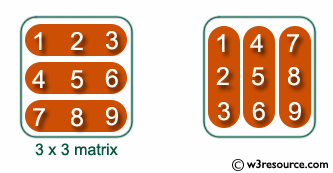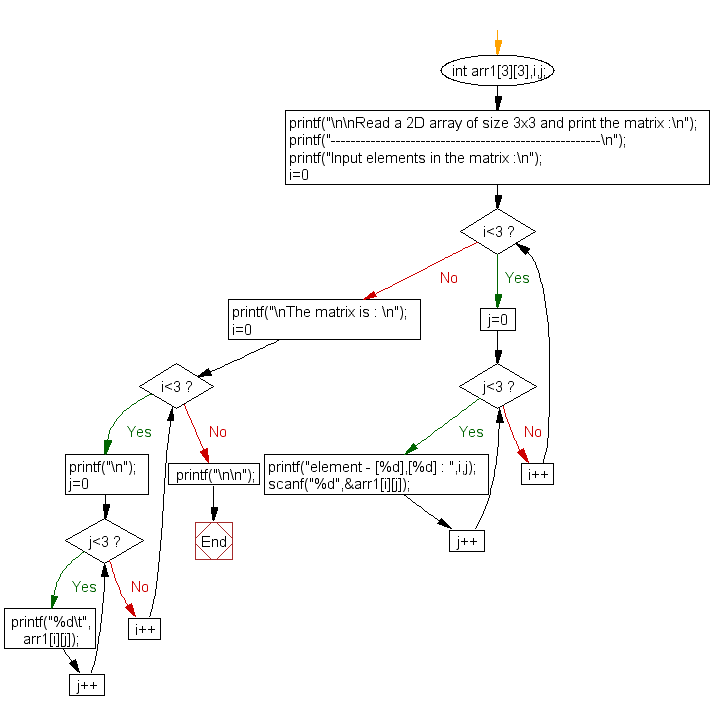﻿ C exercises: Read a 2D array of size 3x3 and print the matrix - w3resource# C Exercises: Read a 2D array of size 3x3 and print the matrix

## C Array: Exercise-18 with Solution

Write a program in C for a 2D array of size 3x3 and print the matrix.

Pictorial Presentation:Sample Solution:

C Code:

``````#include <stdio.h>

void main()
{
int arr1,i,j;

printf("\n\nRead a 2D array of size 3x3 and print the matrix :\n");
printf("------------------------------------------------------\n");

/* Stored values into the array*/
printf("Input elements in the matrix :\n");
for(i=0;i<3;i++)
{
for(j=0;j<3;j++)
{
printf("element - [%d],[%d] : ",i,j);
scanf("%d",&arr1[i][j]);
}
}

printf("\nThe matrix is : \n");
for(i=0;i<3;i++)
{
printf("\n");
for(j=0;j<3;j++)
printf("%d\t",arr1[i][j]);
}
printf("\n\n");
}
```
```

Sample Output:

```Read a 2D array of size 3x3 and print the matrix :
------------------------------------------------------
Input elements in the matrix :
element - , : 1
element - , : 2
element - , : 3
element - , : 4
element - , : 5
element - , : 6
element - , : 7
element - , : 8
element - , : 9

The matrix is :

1       2       3
4       5       6
7       8       9
```

Flowchart:C Programming Code Editor:

Improve this sample solution and post your code through Disqus.

What is the difficulty level of this exercise?

﻿